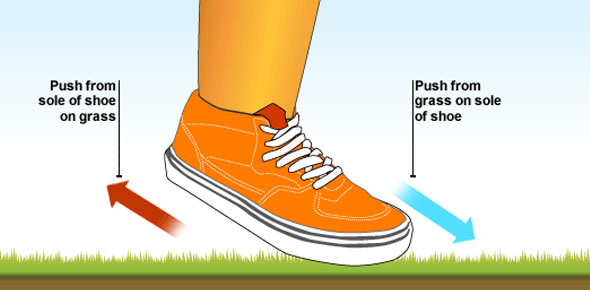# Motion And Speed Trivia Quiz

5 Questions | Attempts: 1276SettingsThis quiz will test whether students understand the concepts behind viewing motion. It will assess if students recognize common units involved with motion. It will also assess whether or not students will be able to calculate the average speed of an object in motion.

• 1.
If a student was walking a distance of 150 meters in 30 seconds, what would that students average speed be?
• A.

50 meters/second

• B.

30 meters/second

• C.

45 meters/second

• D.

35 meters/second

• E.

25 meters/second

• 2.
Watch the first 30 seconds of the video and explain in 3-4 sentences why this shows motion using the vocabulary terms: reference point, reference directions, and position.
• 3.
Explain how you would be able to see these clouds move using vocabulary terms from this chapter.
• 4.
What is the correct equation for average speed?
• A.

Average speed = total time ÷ total distance

• B.

Average speed = total distance ÷ total time

• C.

Average speed = total distance x total time

• D.

Average speed = total distance + total time

• E.

Average speed = total distance - total time

• 5.
A student is traveling with a constant speed of 3 meters/second on their way to science class.  There class is 30 meters away, and they have 15 seconds before the tardy bell rings.  Will they make it to class on time, or get detention for being late?

## Related TopicsBack to top
×

Wait!
Here's an interesting quiz for you.